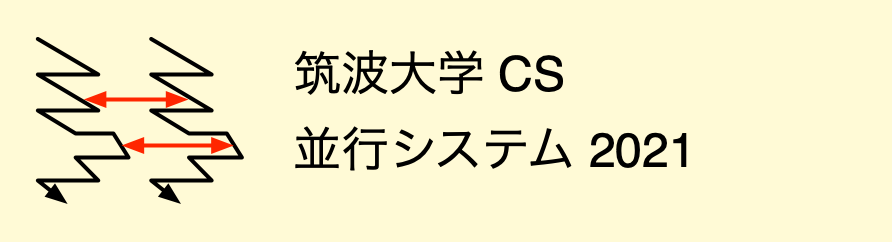# \$BJB9T%7%9%F%`(B(2021\$BG/(B)\$B!?%l%]!<%H(B

## \$B"#O"Mm;v9`(B

• \$BDs=P\$7\$?%l%]!<%H\$O!":FDs=P\$K\$J\$C\$F\$\$\$k\$3\$H\$,\$"\$k!#(B \$B\$=\$N\$h\$&\$J%l%]!<%H\$O!"(BOK \$B\$K\$J\$k\$^\$G=\$@5\$7\$F:FDs=P\$9\$k\$3\$H!#(B Manaba \$B\$N%3!<%9@.@S0lMw(B \$B\$G:FDs=P\$N\$b\$N\$,3NG'\$G\$-\$k!#(B
Submitted reports can be marked as "resubmit". You should rewrite and submit such reports until you see "OK". You can see the status of all your reports at the Manaba page of course grades.
• \$B%l%]!<%H\$r:NE@\$7\$?7k2L!"!V(BOK\$B!W\$H\$J\$C\$F\$\$\$k?M\$O!"%l%]!<%H\$r:FDs=P\$7\$J\$\$(B \$B\$G2<\$5\$\$!#!V:FDs=P!W\$H\$J\$C\$F\$\$\$k\$b\$N\$@\$1!":FDs=P\$7\$F2<\$5\$\$!#(B Manaba \$B\$N%3!<%9@.@S0lMw(B \$B\$G:FDs=P\$N\$b\$N\$,3NG'\$G\$-\$k\$H;W\$\$\$^\$9!#(B You should not resubmit a report if it is marked as "OK". You should rewrite and submit a report when it is marked as "resubmit". You can see the status of all your reports at the Manaba page of course grades.
• \$B2sEz\$O!"9V5AFbMF\$K\$=\$C\$?\$b\$N\$K\$7\$J\$5\$\$!#(BWikipedia \$B\$=\$NB>\$N?.Mj\$G\$-\$J\$\$(B \$B%=!<%9\$+\$iF@\$?>pJs\$r2sEz\$7\$J\$\$\$3\$H!#(B You should find answers from the contents of the class. You should not copy and paste the contents from untested sources, including Wikipedia.
• \$B3F2s\$G!"\$9\$Y\$F\$N2sEz\$r4^\$`%F%-%9%H%U%!%\$%k(B1\$B\$D\$r:n@.\$7!"(B Manaba \$B\$N!V%l%]!<%H!W%?%V\$KDs=P\$7\$J\$5\$\$!#(B For each class, you must make a single text file and submit it to the Manaba report tab.
[2021/06/18 \$BDI2C(B]
• \$B4|Kv;n83\$O9T\$\$\$^\$;\$s!#4|Kv%l%]!<%H\$b\$"\$j\$^\$;\$s!#Kh2s\$N%l%]!<%H\$G@.(B \$B@S\$rIU\$1\$^\$9!#(B There is no final exam and no final report. Grade are determined by individual class reports.
• \$B%l%]!<%H=P\$7\$F\$\$\$J\$\$?M\$O(B Web \$B%Z!<%8\$+\$i=P\$7\$F\$/\$@\$5\$\$!#(B \$B2a5n\$N%l%]!<%H\$rDy@Z2a\$.\$?8e\$G\$bDs=P\$G\$-\$^\$9!#(B You must submit reports via the Web page. You can submit past reports after the deadlines.
• \$B%l%]!<%H\$O!"(B6\$B7n(B30\$BF|(B 17:00 \$B\$^\$G\$KA4ItDs=P\$7\$F\$/\$@\$5\$\$!#(B You must submit all the reports by June 30 17:00.
• \$B0lIt!":FDs=P\$K\$J\$C\$F\$\$\$k\$b\$N\$,\$"\$j\$^\$9!#(B You may have some reports to post again.
• Twins\$B\$K\$"\$k2A%"%s%1!<%H(B \$B\$K2sEz\$*4j\$\$\$7\$^\$9!#(B \$B2sEz4|4V\$O!"(B6\$B7n(B17\$BF|\$+\$i(B7\$B7n(B14\$BF|\$G\$9!#(B Please answer the course evaluation questionnaire in Twins between June 17 and July 14.

## \$B"#%l%]!<%H2]Bj(B

### \$B"#2]Bj(B1

\$BN}=,LdBj(B1\$B\$N(B (101)\$B\$+\$i(B(105)\$B\$r\$9\$Y\$F2sEz\$7\$J\$5\$\$!#(B Answer *all* the exercises (101) to (105).

### \$B"#2]Bj(B2

\$BN}=,LdBj(B2\$B\$N(B (210)\$B\$H(B(211)\$B\$K2sEz\$7\$J\$5\$\$!#(B Answer the exercises (210) and (211).

### \$B"#2]Bj(B3

\$BN}=,LdBj(B3\$B\$N(B (301)\$B\$+\$i(B(304)\$B\$r\$9\$Y\$F2sEz\$7\$J\$5\$\$!#(B Answer *all* the exercises (301) to (304).

### \$B"#2]Bj(B4

\$BN}=,LdBj(B4\$B\$N(B (401)\$B\$+\$i(B(404)\$B\$r\$9\$Y\$F2sEz\$7\$J\$5\$\$!#(B Answer *all* the exercises (401) to (404).

### \$B"#2]Bj(B5

\$BN}=,LdBj(B5\$B\$N(B (506)\$B\$H(B(507)\$B\$K2sEz\$7\$J\$5\$\$!#(B Answer the exercises (506) and (507).

\$B2sEz\$r4^\$`!V%F%-%9%H!W%U%!%\$%k(B1\$B\$D\$r:n@.\$7!"0J2<\$NJ}K!\$GDs=P\$7\$J\$5\$\$!#(B Make a single *text* file and submit it as described below.

[2021/04/12 \$BDI2C(B] \$B

### \$B"#2]Bj(B6

\$BN}=,LdBj(B6\$B\$N(B (601)\$B\$+\$i(B(603)\$B\$r\$9\$Y\$F2sEz\$7\$J\$5\$\$!#(B Answer *all* the exercises (601) to (603).

### \$B"#2]Bj(B7

\$BN}=,LdBj(B7\$B\$N(B (707)\$B\$K2sEz\$7\$J\$5\$\$!#(B Answer the exercise (707).

### \$B"#2]Bj(B8

\$BN}=,LdBj(B8\$B\$N(B (801)\$B\$+\$i(B(804)\$B\$r\$9\$Y\$F2sEz\$7\$J\$5\$\$!#(B Answer *all* the exercises (801) to (804).

### \$B"#2]Bj(B9

\$BN}=,LdBj(B9\$B\$N(B (901)\$B\$+\$i(B(903)\$B\$r\$9\$Y\$F2sEz\$7\$J\$5\$\$!#(B Answer *all* the exercises (901) to (903).

\$B2sEz\$r4^\$`!V%F%-%9%H!W%U%!%\$%k(B1\$B\$D\$r:n@.\$7!"0J2<\$NJ}K!\$GDs=P\$7\$J\$5\$\$!#(B Make a single *text* file and submit it as described below.

### \$B"#2]Bj(B10

\$BN}=,LdBj(B10\$B\$N(B (1001)\$B\$+\$i(B(1003)\$B\$r\$9\$Y\$F2sEz\$7\$J\$5\$\$!#(B Answer *all* the exercises (1001) to (1003).

[2021/06/26 \$BDI2C(B] 25\$BF|\$^\$G\$KDs=P\$5\$l\$?%l%]!<%H\$O\$9\$Y\$F:NE@\$7\$?\$D\$b\$j\$G\$9!#(B

## \$B"#%l%]!<%H\$NDs=PJ}K!(B

\$B\$^\$:!"

First, you should make a single plain text file. You should not use Markdown, HTML, or other markup languages. We accept the following character codes.

• JIS\$B!"(BShift-JIS\$B!"(BEUC
• UTF-8
• US-ASCII
PDF, RTF, \$B%o!<%I%W%m%;%C%5\$NJ8=q%U%!%\$%k\$r)\$a\$k!#(B

We do not accept PDF, RTF, or word processor documents. You should not compress the text file with ZIP, tar, or gzip. You may want to download and check the file.

```\$B3X@RHV9f(B(Student ID number): 202167890
\$BL>A0(B(Name): \$BL>A0(B(\$B4A;z\$,\$"\$l\$P4A;z!#\$J\$1\$l\$P1Q8l!#(BKanji or English name)
\$B2]BjHV9f(B(Assignment Number)\$B!'(BN
\$BBjL>(B(Subject)\$B!'(Bsubject

\$B!cFbMF!d(B
```
\$BK\J8\$N@hF,\$K3X@RHV9f\$HL>A0!J4A;z\$NL>A0\$,\$"\$k?M\$O!"4A;z\$G!K\$r=q\$-\$J\$5\$\$!#(B \$B!V2]BjHV9f!W\$H\$O!"9V5A\$N2s?t\$G\$"\$k!#!VBjL>!W\$K\$O!"EE;R%a!<%k\$N(B Subject: \$B\$HF1MM\$K!"8e\$G8+JV\$7\$?;~\$KFbMF\$r;W\$\$=P\$;\$k\$h\$&\$KFbMF\$KB(\$7\$?\$b(B \$B\$N\$r\$D\$1\$k!#!V2]Bj(B1\$B!W\$N\$h\$&\$J(B Subject: \$B\$OITE,@Z\$G\$"\$k!#(B

You should write your student ID number and names (and names in kanji if you have). The assignment number is equal to the class number. The subject should be the one in email, and you can remember the file after you read it later. A wrong subject is "Assignment 1".

\$B!cFbMF!d\$O!"F|K\8l!"\$^\$?\$O1Q8l\$G=q\$-\$J\$5\$\$!#J8>O\$K\$O!"=R8l\$rIU\$1\$k!#(B \$BBN8@;_\$a\$O!";H\$C\$F\$O\$J\$i\$J\$\$!#C1\$K%W%m%0%i%`\$r4^\$a\$k\$N\$G\$O\$J\$/!"!V0J2<(B \$B\$K!{!{\$N%W%m%0%i%`\$r<(\$9!W\$H=q\$/\$3\$H!#!cFbMF!d\$K\$O!"%W%m%0%i%`\$@\$1\$G\$J(B \$B\$/!"

You should use Japanese or English to write the report. A sentence should have a predicate verb. You should not use taigendome (ending a poem with a noun). When you show a program, you should write "The program of XXX follows". The contents must include the execution results (input and output), and descriptions of them.

\$B:n@.\$7\$?%U%!%\$%k\$r!"(B Manaba \$B\$N%l%]!<%H%?%V(B \$B\$+\$iEj9F\$9\$k!#(B

You can submit the report with the report tab in Manaba.

\$B%l%]!<%H\$r:FDs=P\$9\$k;~\$K\$O!"\$I\$NItJ,\$r=\$@5\$7\$?\$N\$+\$,4JC1\$K\$o\$+\$k\$h\$&\$K(B \$B@bL@\$7\$J\$5\$\$!#(B

When you resubmit a report, you should clarify the differences from the previous report.

\$BDs=P\$7\$?%l%]!<%H\$O!"9V5A\$,=*\$k\$^\$GJ]B8\$7\$J\$5\$\$!#(B

You should keep the report files while you are taking this class.

## \$B"#%+%l%s%@!<(B

```
April                  May                   June
Su Mo Tu We Th Fr Sa  Su Mo Tu We Th Fr Sa  Su Mo Tu We Th Fr Sa
1  2  3                     1         1  2  3  4  5
4  5  6  7  8  9 10   2  3  4  5  6  7  8   6  7  8  9 10 11 12
11 12 13 14 15 16 17   9 10 11 12 13 14 15  13 14 15 16 17 18 19
18 19 20 21 22 23 24  16 17 18 19 20 21 22  20 21 22 23 24 25 26
25 26 27 28 29 30     23 24 25 26 27 28 29  27 28 29 30
30 31

```

## \$B"#9V5A\$N%Z!<%8\$XLa\$k(B

Last updated: 2021/06/26 12:32:47
Yasushi Shinjo / <yas@cs.tsukuba.ac.jp>